# RD Sharma Solutions for Class 8 Math Chapter 4 - Cubes and Cube Roots (Part-3) Notes | Study RD Sharma Solutions for Class 8 Mathematics - Class 8

## Class 8: RD Sharma Solutions for Class 8 Math Chapter 4 - Cubes and Cube Roots (Part-3) Notes | Study RD Sharma Solutions for Class 8 Mathematics - Class 8

The document RD Sharma Solutions for Class 8 Math Chapter 4 - Cubes and Cube Roots (Part-3) Notes | Study RD Sharma Solutions for Class 8 Mathematics - Class 8 is a part of the Class 8 Course RD Sharma Solutions for Class 8 Mathematics.
All you need of Class 8 at this link: Class 8

#### (i) We have: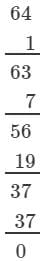Subtraction is performed 4 times.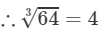(ii) We have: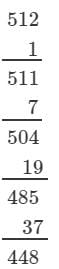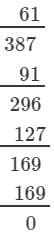Subtraction is performed 8 times.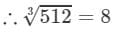(iii) We have: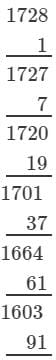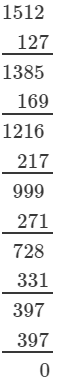Subtraction is performed 12 times.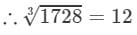#### Answer 2: (i) We have: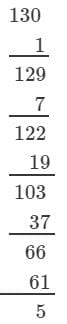The next number to be subtracted is 91, which is greater than 5.
130 is not a perfect cube.

(ii) We have: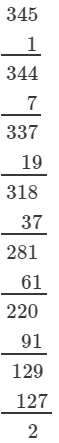The next number to be subtracted is 161, which is greater than 2.
345 is not a perfect cube.

(iii) We have: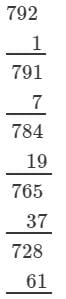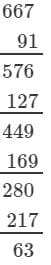The next number to be subtracted is 271, which is greater than 63.
792 is not a perfect cube.

(iv) We have: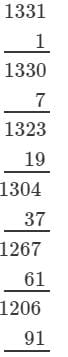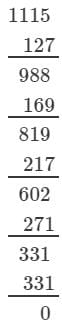The subtraction is performed 11 times.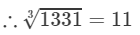Thus, 1331 is a perfect cube.

#### Answer 3: (i) We have: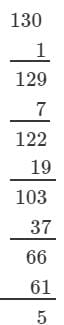The next number to be subtracted is 91, which is greater than 5.
130 is not a perfect cube.

However, if we subtract 5 from 130, we will get 0 on performing successive subtraction and the number will become a perfect cube.
If we subtract 5 from 130, we get 125. Now, find the cube root using successive subtraction.
We have: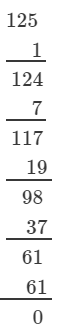The subtraction is performed 5 times.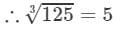Thus, it is a perfect cube.

(ii) We have: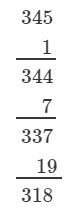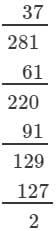The next number to be subtracted is 161, which is greater than 2.
345 is not a perfect cube.
However, if we subtract 2 from 345, we will get 0 on performing successive subtraction and the number will become a perfect cube.
If we subtract 2 from 345, we get 343. Now, find the cube root using successive subtraction.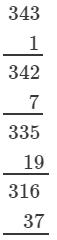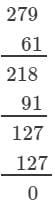The subtraction is performed 7 times.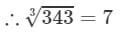Thus, it is a perfect cube.

(iii) We have: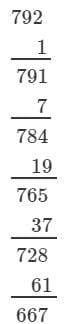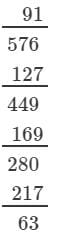The next number to be subtracted is 271, which is greater than 63.
792 is not a perfect cube.
However, if we subtract 63 from 792, we will get 0 on performing successive subtraction and the number will become a perfect cube.
If we subtract 63 from 792, we get 729. Now, find the cube root using the successive subtraction.
We have: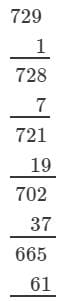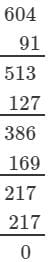The subtraction is performed 9 times.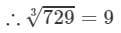Thus, it is perfect cube.

#### Answer 4: (i) Cube root using units digit:Let us consider 343.The unit digit is 3; therefore, the unit digit in the cube root of 343 is 7.There is no number left after striking out the units, tens and hundreds digits of the given number; therefore, the cube root of 343 is 7.Hence,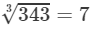(ii) Cube root using units digit:
Let us consider 2744.
The unit digit is 4; therefore, the unit digit in the cube root of 2744 is 4.
After striking out the units, tens and hundreds digits of the given number, we are left with 2.
Now, 1 is the largest number whose cube is less than or equal to 2.
Therefore, the tens digit of the cube root of 2744 is 1.
Hence,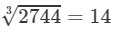(iii) Cube root using units digit:
Let us consider 4913.
The unit digit is 3; therefore, the unit digit in the cube root of 4913 is 7.
After striking out the units, tens and hundreds digits of the given number, we are left with 4.
Now, 1 is the largest number whose cube is less than or equal to 4.
Therefore, the tens digit of the cube root of 4913 is 1.

Hence,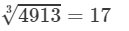(iv) Cube root using units digit:
Let us consider 1728.
The unit digit is 8; therefore, the unit digit in the cube root of 1728 is 2.
After striking out the units, tens and hundreds digits of the given number, we are left with 1.
Now, 1 is the largest number whose cube is less than or equal to 1.
Therefore, the tens digit of the cube root of 1728 is 1.
Hence,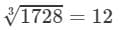(v) Cube root using units digit:
Let us consider 35937.
The unit digit is 7; therefore, the unit digit in the cube root of 35937 is 3.
After striking out the units, tens and hundreds digits of the given number, we are left with 35.
Now, 3 is the largest number whose cube is less than or equal to 35 ( 33<35<4333<35<43).
Therefore, the tens digit of the cube root of 35937 is 3.
Hence,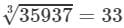(vi) Cube root using units digit:
Let us consider the number 17576.
The unit digit is 6; therefore, the unit digit in the cube root of 17576 is 6.
After striking out the units, tens and hundreds digits of the given number, we are left with 17.
Now, 2 is the largest number whose cube is less than or equal to 17 (23<17<3323<17<33).
Therefore, the tens digit of the cube root of 17576 is 2.
Hence,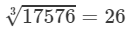(vii) Cube root by factors:
On factorising 134217728 into prime factors, we get:
134217728=2×2×2×2×2×2×2×2×2×2×2×2×2×2×2×2×2×2×2×2×2×2×2×2×2×2×2

On grouping the factors in triples of equal factors, we get:
134217728={2×2×2}×{2×2×2}×{2×2×2}×{2×2×2}×{2×2×2}×{2×2×2}×{2×2×2}×{2×2×2}×{2×2×2}
Now, taking one factor from each triple, we get: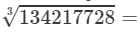2×2×2×2×2×2×2×2×2=5121342177283=2×2×2×2×2×2×2×2×2=512

(viii) Cube root by factors:
On factorising 48228544 into prime factors, we get:

48228544=2×2×2×2×2×2×7×7×7×13×13×1348228544=2×2×2×2×2×2×7×7×7×13×13×13
On grouping the factors in triples of equal factors, we get:
48228544={2×2×2}×{2×2×2}×{7×7×7}×{13×13×13}48228544=2×2×2×2×2×2×7×7×7×13×13×13
Now, taking one factor from each triple, we get: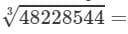2×2×7×13=364

(ix) Cube root by factors:
On factorising 74088000 into prime factors, we get:
74088000=2×2×2×2×2×2×3×3×3×5×5×5×7×7×774088000=2×2×2×2×2×2×3×3×3×5×5×5×7×7×7
On grouping the factors in triples of equal factors, we get:
74088000={2×2×2}×{2×2×2}×{3×3×3}×{5×5×5}×{7×7×7}74088000=2×2×2×2×2×2×3×3×3×5×5×5×7×7×7

Now, taking one factor from each triple, we get: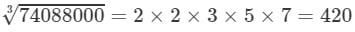(x) Cube root using units digit:

Let is consider 157464.
The unit digit is 4; therefore, the unit digit in the cube root of 157464 is 4.
After striking out the units, tens and hundreds digits of the given number, we are left with 157.
Now, 5 is the largest number whose cube is less than or equal to 157 (53<157<6353<157<63).
Therefore, the tens digit of the cube root 157464 is 5.
Hence,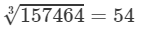(xi) Cube root by factors:
On factorising 1157625 into prime factors, we get:
1157625=3×3×3×5×5×5×7×7×71157625=3×3×3×5×5×5×7×7×7
On grouping the factors in triples of equal factors, we get:
1157625={3×3×3}×{5×5×5}×{7×7×7}1157625=3×3×3×5×5×5×7×7×7
Now, taking one factor from each triple, we get: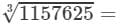3×5×7=10511576253=3×5×7=105

(xii) Cube root by factors:
On factorising 33698267 into prime factors, we get:
33698267=17×17×17×19×19×1933698267=17×17×17×19×19×19
On grouping the factors in triples of equal factors, we get:
33698267={17×17×17}×{19×19×19}33698267=17×17×17×19×19×19
Now, taking one factor from each triple, we get: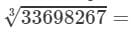17×19=323336982673=17×19=323

#### Answer 7: On factorising 8192 into prime factors, we get:8192=2×2×2×2×2×2×2×2×2×2×2×2×28192=2×2×2×2×2×2×2×2×2×2×2×2×2On grouping the factors in triples of equal factors, we get:8192={2×2×2}×{2×2×2}×{2×2×2}×{2×2×2}×28192=2×2×2×2×2×2×2×2×2×2×2×2×2It is evident that the prime factors of 8192 cannot be grouped into triples of equal factors such that no factor is left over. Therefore, 8192 is not a perfect cube. However, if the number is divided by 2, the factors can be grouped into triples of equal factors such that no factor is left over.Hence, the number 8192 should be divided by 2 to make it a perfect cube.Also, the quotient is given as: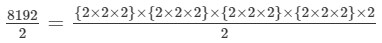⇒4096={2×2×2}×{2×2×2}×{2×2×2}×{2×2×2}

To get the cube root of the quotient 4096, take one factor from each triple. We get:

Cube root = 2×2×2×2=162×2×2×2=16

Hence, the required numbers are 2 and 16.

#### Answer 8: Let the numbers be x, 2x and 3x.

Therefore

x3+(2x)3+(3x)3=98784x3+8x3+273=9878436x3=98784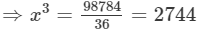x3=2744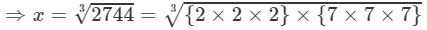=2×7=14

Hence, the numbers are 14, (2×14=282×14=28) and (3×14=423×14=42).

#### 9261000=2×2×2×3×3×3×5×5×5×7×7×7={2×2×2}×{3×3×3}×{5×5×5}×{7×7×7}9261000 could be written as a triples of equal factors; therefore, we get:Cube root = 2×3×5×7=2102×3×5×7=210Therefore

s3=9261000s=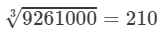Hence, the length of the side of cube is 210 m.

The document RD Sharma Solutions for Class 8 Math Chapter 4 - Cubes and Cube Roots (Part-3) Notes | Study RD Sharma Solutions for Class 8 Mathematics - Class 8 is a part of the Class 8 Course RD Sharma Solutions for Class 8 Mathematics.
All you need of Class 8 at this link: Class 8Use Code STAYHOME200 and get INR 200 additional OFF

## RD Sharma Solutions for Class 8 Mathematics

88 docs

Track your progress, build streaks, highlight & save important lessons and more!

,

,

,

,

,

,

,

,

,

,

,

,

,

,

,

,

,

,

,

,

,

;